# Partial Orderings Aaron Bloomfield CS 202 Epp section

• Slides: 14
Download presentation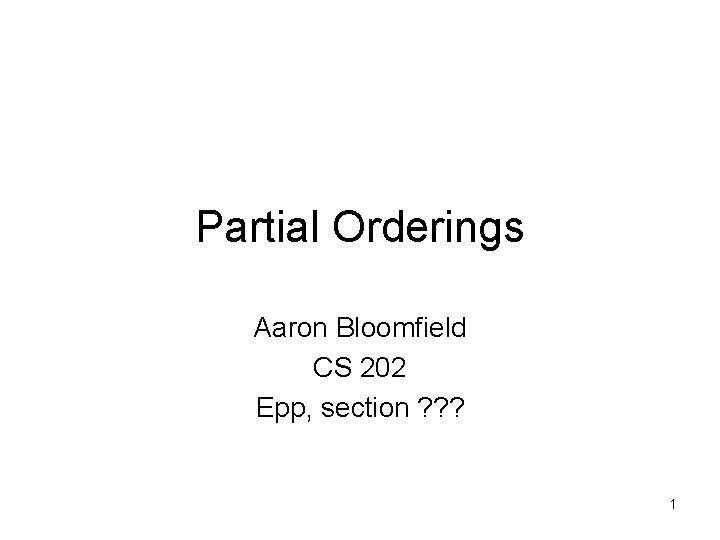Partial Orderings Aaron Bloomfield CS 202 Epp, section ? ? ? 1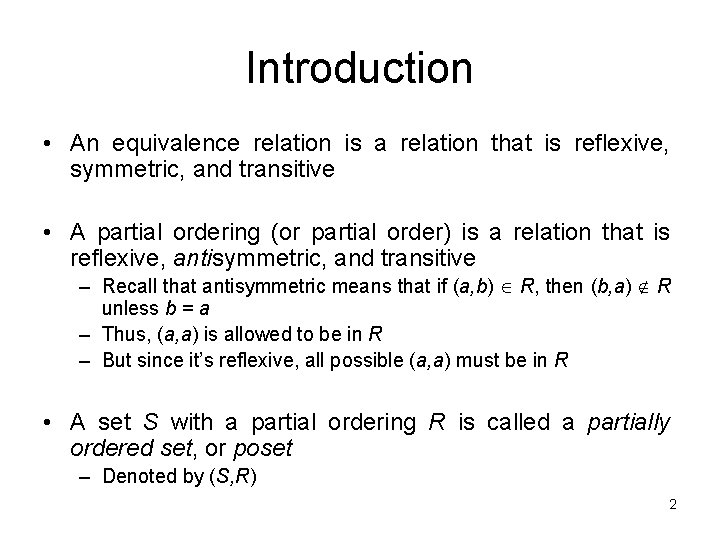Introduction • An equivalence relation is a relation that is reflexive, symmetric, and transitive • A partial ordering (or partial order) is a relation that is reflexive, antisymmetric, and transitive – Recall that antisymmetric means that if (a, b) R, then (b, a) R unless b = a – Thus, (a, a) is allowed to be in R – But since it’s reflexive, all possible (a, a) must be in R • A set S with a partial ordering R is called a partially ordered set, or poset – Denoted by (S, R) 2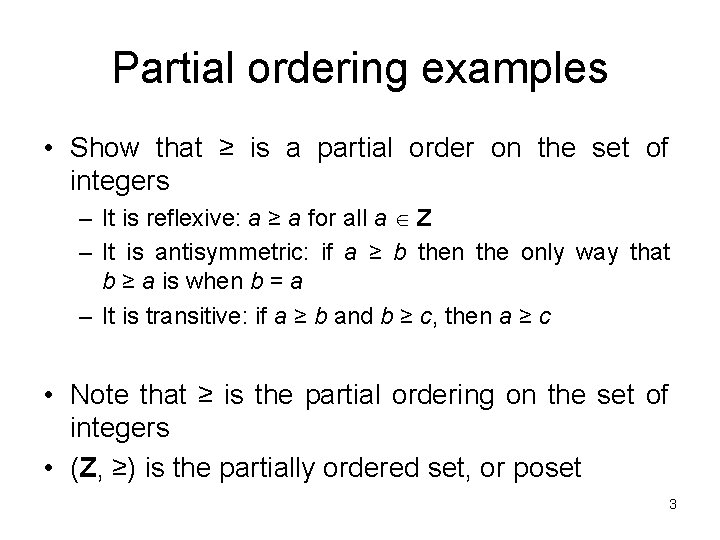Partial ordering examples • Show that ≥ is a partial order on the set of integers – It is reflexive: a ≥ a for all a Z – It is antisymmetric: if a ≥ b then the only way that b ≥ a is when b = a – It is transitive: if a ≥ b and b ≥ c, then a ≥ c • Note that ≥ is the partial ordering on the set of integers • (Z, ≥) is the partially ordered set, or poset 3Symbol usage • The symbol is used to represent any relation when discussing partial orders – – Not just the less than or equals to relation Can represent ≤, ≥, , etc Thus, a b denotes that (a, b) R The poset is (S, ) • The symbol is used to denote a b but a ≠ b – If represents ≥, then represents > • Fonts for this lecture set (specifically for the and symbols) are available on the course website 4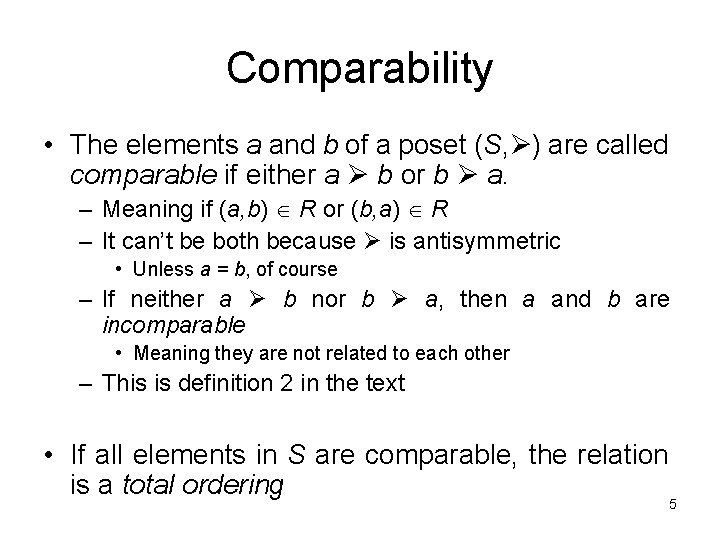Comparability • The elements a and b of a poset (S, ) are called comparable if either a b or b a. – Meaning if (a, b) R or (b, a) R – It can’t be both because is antisymmetric • Unless a = b, of course – If neither a b nor b a, then a and b are incomparable • Meaning they are not related to each other – This is definition 2 in the text • If all elements in S are comparable, the relation is a total ordering 5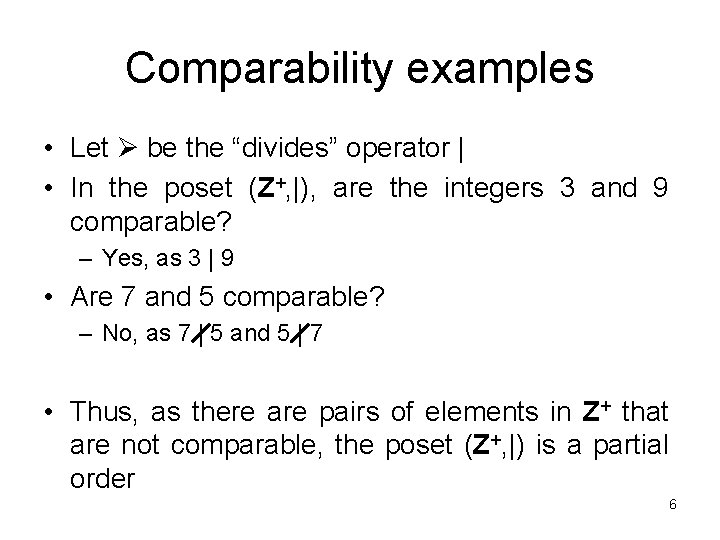Comparability examples • Let be the “divides” operator | • In the poset (Z+, |), are the integers 3 and 9 comparable? – Yes, as 3 | 9 • Are 7 and 5 comparable? – No, as 7 | 5 and 5 | 7 • Thus, as there are pairs of elements in Z+ that are not comparable, the poset (Z+, |) is a partial order 6Comparability examples • Let be the less than or equals operator ≤ • In the poset (Z+, ≤), are the integers 3 and 9 comparable? – Yes, as 3 ≤ 9 • Are 7 and 5 comparable? – Yes, as 5 ≤ 7 • As all pairs of elements in Z+ are comparable, the poset (Z+, ≤) is a total order – a. k. a. totally ordered poset, linear order, chain, etc. 7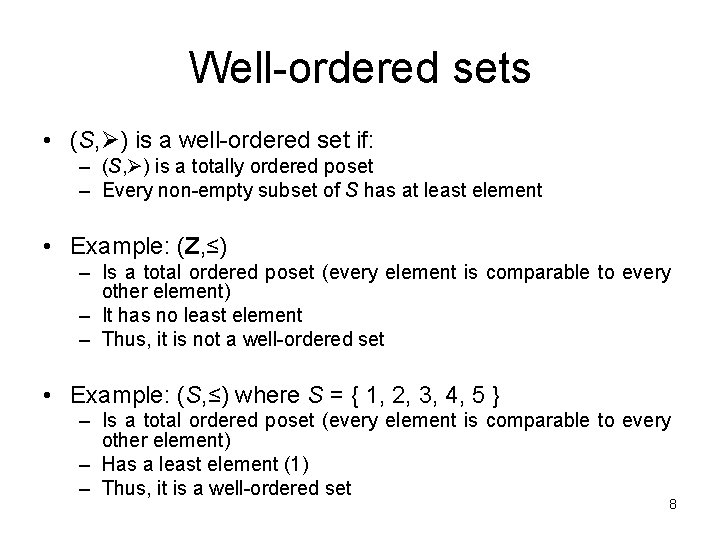Well-ordered sets • (S, ) is a well-ordered set if: – (S, ) is a totally ordered poset – Every non-empty subset of S has at least element • Example: (Z, ≤) – Is a total ordered poset (every element is comparable to every other element) – It has no least element – Thus, it is not a well-ordered set • Example: (S, ≤) where S = { 1, 2, 3, 4, 5 } – Is a total ordered poset (every element is comparable to every other element) – Has a least element (1) – Thus, it is a well-ordered set 8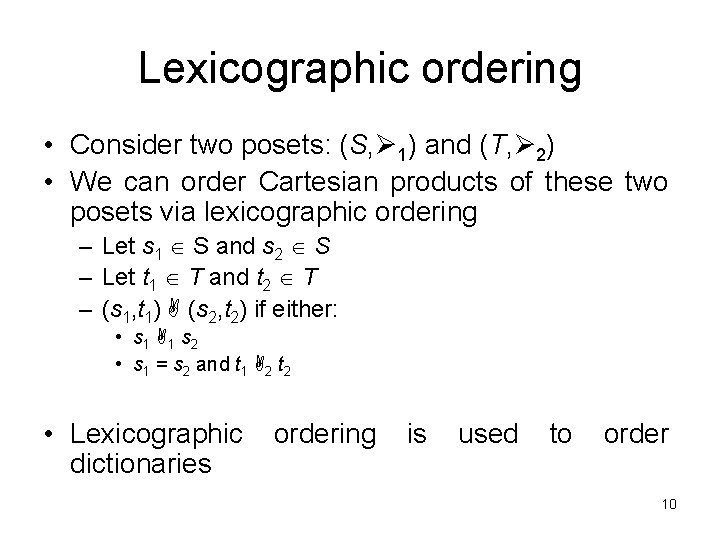Lexicographic ordering • Consider two posets: (S, 1) and (T, 2) • We can order Cartesian products of these two posets via lexicographic ordering – Let s 1 S and s 2 S – Let t 1 T and t 2 T – (s 1, t 1) (s 2, t 2) if either: • s 1 1 s 2 • s 1 = s 2 and t 1 2 t 2 • Lexicographic dictionaries ordering is used to order 10Lexicographic ordering • Let S be the set of word strings (i. e. no spaces) • Let T bet the set of strings with spaces • Both the relations are alphabetic sorting – We will formalize alphabetic sorting later • Thus, our posets are: (S, ) and (T, ) • Order (“run”, “noun: to…”) and (“set”, “verb: to…”) – As “run” “set”, the “run” Cartesian product comes before the “set” one • Order (“run”, “noun: to…”) and (“run”, “verb: to…”) – Both the first part of the Cartesian products are equal – “noun” is first (alphabetically) than “verb”, so it is ordered first 11Lexicographic ordering • We can do this on more than 2 -tuples • (1, 2, 3, 5) (1, 2, 4, 3) – When is ≤ 12Lexicographic ordering • Consider the two strings a 1 a 2 a 3…am, and b 1 b 2 b 3…bn • Here follows the formal definition for lexicographic ordering of strings • If m = n (i. e. the strings are equal in length) – (a 1, a 2, a 3, …, am) (b 1, b 2, b 3, …, bn) using the comparisons just discussed – Example: “run” “set” • If m ≠ n, then let t be the minimum of m and n – Then a 1 a 2 a 3…am, is less than b 1 b 2 b 3…bn if and only if either of the following are true: – (a 1, a 2, a 3, …, at) (b 1, b 2, b 3, …, bt) • Example: “run” “sets” (t = 3) – (a 1, a 2, a 3, …, at) = (b 1, b 2, b 3, …, bt) and m < n • Example: “run” “running” 13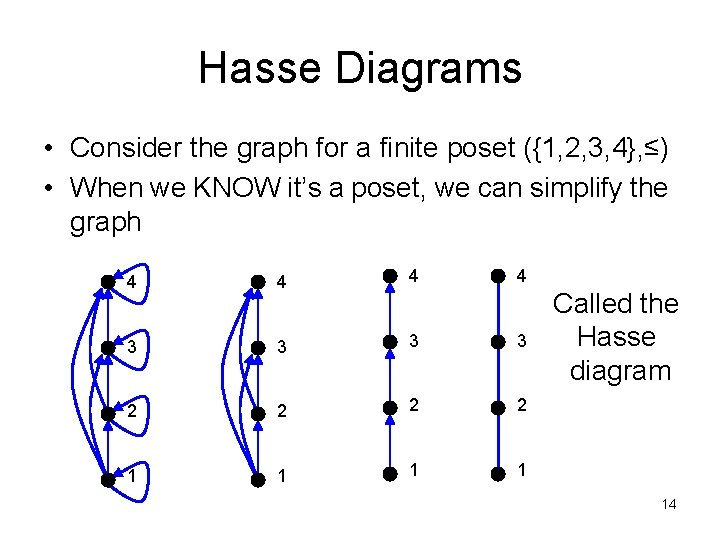Hasse Diagrams • Consider the graph for a finite poset ({1, 2, 3, 4}, ≤) • When we KNOW it’s a poset, we can simplify the graph 4 4 3 3 2 2 1 1 Called the Hasse diagram 14Hasse Diagram • For the poset ({1, 2, 3, 4, 6, 8, 12}, |) 15